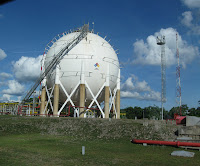# EROSIONAL SPEED OF GAS – CALCULATION OF TRANSMISSIONWhen a fluid flows through a pipe at a high speed can cause vibration and erosion in the pipe, which erodes the wall of the pipe. If the gas velocity exceeds the speed erosional calculated for the pipe, the erosion of the pipe wall is incrementacla at rates that can shorten the life of the pipe significantly. Therefore it is always necessary to control the speed of the gas transmission line to prevent the excess of the limit.

The erosional velocity for compressible fluids is expressed as:

Where:

Erosional velocity Ve = Ft / sec;
p = gas density, lbm / ft;
And C is a constant-defined as 75 <150. c = "100."> Donde:

Where:

Erosional velocity Ve = Ft / sec;